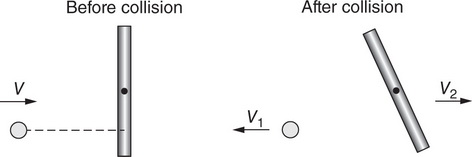# AP Physics 1 Question 176: Answer and Explanation

### Test Information

Question: 176

6.Question below refers to the following information:

A small ball of mass m moving to the right at speed v collides with a stationary rod, as shown in the figure. After the collision, the ball rebounds to the left with speed v1, while the rod’s center of mass moves to the left at speed v2. The rod also rotates counterclockwise.

Which of the following equations determines the rod’s change in angular momentum about its center of mass during the collision?

• A. where I is the rod’s rotational inertia about its center of mass, and ω is its angular speed after collision.
• B. Iv2/r, where I is the rod’s rotational inertia about its center of mass, and r is half the length of the rod.
• C. mv1d, where d is the distance between the line of the ball’s motion and the rod’s center of mass.
• D. mv1r, where r is half the length of the rod.